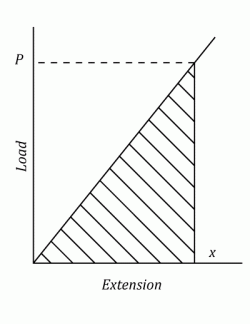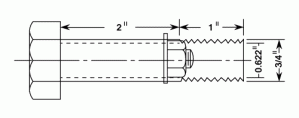I have forgotten

•http://facebook.com/
•https://www.google.com/accounts/o8/id
•https://me.yahoo.com

# Direct Stress

Resilience and Direct Stress in bars, both composite and of varying cross section.View other versions (5)

## Introduction

A structural member is said to be under Direct Stress when the loads acting on it are either compressive or tensile. Any other forms of loading, including bending and shear, cannot be said to cause Direct Stress.

This section highlights the Direct Stress and the resilience in bars of varying cross section as well as in compound bars; made with more than one material, each having its coefficient of thermal expansion.

## Strain Energy, ResilienceWhen either a Tensile or Compressive Load is applied to a bar, there will be a change in length, which for a material that obeys Hooke's law, is proportional to the load.

The Strain Energyof the bar is defined as the work done by the load in altering the length (i.e. Producing Strain).

For a gradually applied or "Static" load the work done is represented by the shaded area on the diagram.The Strain Energy can be expressed in terms of the Stress and dimensions. For a bar of uniform cross section and length.Tension is the magnitude of the pulling force exerted by a string, cable, chain, or similar object on another object. It is the opposite of compression. As tension is the magnitude of a force, it is measured in newtons (or sometimes pounds-force) and is always measured parallel to the string on which it applies.

Compression is the result of the subjection of a material to compressive stress, which results in reduction of volume as compared to an uncompressed but otherwise identical state.

Butis the volume of the bar and can be expressed in words as:

The Strain Energy per unit volume (Usually called Resilience) in either simple Tension or Compression is :• Proof Resilience is the value at the elastic limit or for non-ferrous materials, the Proof Stress.
• Strain Energy is always a positive quantity and is expressed in units of work. (In the imperial system in.lb. ; ft.lbs or in.tons)

Example:
[imperial]
##### Example - Example 1
Problem
Calculate the Strain Energy of the following Bolt which is under a Tensile Load of .Show that the Strain Energy is increased when the bolt is under maximum Stress, if the shank of the Bolt is turned down to the root diameter of the thread.

Workings
It is normal practice to assume that the load is distributed evenly over the core of the screwed portion ( i.e. In this case the core diameter is and the Area of the core is . The Cross sectional Area of the shank is

The Stress in the screwed portion is given by:

(Note )

The Stress in the Shank is given by:

Thus, the Total Strain Energy is given by:

If the shank is now reduced in diameter to the Stress in the bolt will be constant and have a value of and the Strain Energy will be given by:
Solution
• The Strain Energy is

If a weightfalls through a heightonto a collar attached at one end of a uniform bar (See Diagram),

the bar will extend. The extent of this extension will be greater than if the load had been gradually applied.

This must be so since the weight will have Kinetic Energy at the point of impact and this energy is absorbed by an increase in the strain energy of the bar. Assuming that the bar does not fail the weight will oscillate about and finally come to rest at the Normal equilibrium position.

In the diagram the maximum extension of the bar is shown as.is the equivalent static or gradually applied load which would produce an extension of.

Velocity is the measurement of the rate and direction of change in the position of an object. It is a vector physical quantity; both magnitude and direction are required to define it.

The kinetic energy of an object is the energy which it possesses due to its motion. It is defined as the work needed to accelerate a body of a given mass from rest to its stated velocity.

The Strain Energy in the bar at the point of maximum extension isIf the loss of energy at Impact is neglected then the following equation can be written:

Loss of Potential Energy by the Weight = Increase in Strain Energy in the Bar i.e.(see equations (2) and (3))

Hence,Rearranging and multiplying through bya quadratic inis produced. i.e.Solving and discarding the negative root:Using the resulting value forit is now possible to calculate both the value of the maximum extensionand the resulting Direct Stress.

The particular case where(i.e. the Load is suddenly applied gives the valuei.e. The Stress produced by a suddenly applied load is twice the Static Stress.

The above simple analysis assumes that the whole of the rod attains the same value of maximum Stress at the same moment. This is however not strictly true. A wave of stress is set up by the Impact which is propogated along the rod.

The actual maximum Stress set up will then depend upon the dimensions of the rod, its density and the velocity of the load at impact. Usually this approximate analysis gives results that err on the "Safe side" but this is not always the case.

Example:
[imperial]
##### Example - Example 2
Problem
Referring to the diagram shown above, let a weight of fall a distance onto a collar at the end of a diameter steel rod.

If the rod is long what is the maximum Stress set up.
Workings
Using the results of equation (3)

Note the length of the bar is

i.e. Although the Load only dropped 2 inches ( Approx 5 cms.) the maximum Stress was nearly 64 times the "Static" Stress.
Solution
• The maximum Stress was nearly 64 times the "Static" Stress.

Weight is the force that gravitation exerts upon a body, equal to the mass of the body times the local acceleration of gravity: commonly taken, in a region of constant gravitational acceleration, as a measure of mass.

It is usual to assume that the load is uniformly distributed over the cross-section and that therefore the Stress is inversely proportional to the area.

The load may also vary as in the case of a column where its own weight needs to be taken into account and of course the case of inertia loadings on members in motion.
Example:
[imperial]
##### Example - Example 5
Problem
A rod of length tapers uniformly from a diameter at one end to a diameter at the other.

Find the extension caused by an axial load .
Workings
At a distance from the small end the diameter is given by:

At the load will extend a short length by:

And for the whole rod the extension is given by:
Solution
• The extension is

## Compound Bars

Any Tensile or Compressive member which consists of two or more bars or tubes in parallel is called a Compound Bar.
The bars are usually of different materials. The method of analysis is shown in the following examples.
Example:
[imperial]
##### Example - Example 8
Problem
A compound bar is made up of a rod of area and modulus and a tube of equal length of area and modulus .

If a compressive load is applied to the Compound Bar find how the Load is shared.
Workings
Since the rod and tube are of the same length and must remain so, the strain in each must be the same. The total load carried is and we can assume that it is shared as and .

From the Strain equation (See Direct Stress Introduction equation (2))

And by equilibrium,

Combing equations (1) and (2),

And

Re-arranging,

Also,
Solution
• The load is shared as and , where and

## Temperature Stresses

If a Compound bar is made of several different materials with different coefficients of thermal expansion, then if it is subjected to a change in temperature, then the different parts will tend to expand by different amounts.

Temperature is a measurement of the average kinetic energy of the molecules in an object or system and can be measured with a thermometer or a calorimeter. It is a means of determining the internal energy contained within the system.

If the parts are constrained to remain together, then the actual change in length must be the same for each. This change will be the result ( taking into account positive and negative Strains) of the effects due to both temperature and Stress conditions.

Example:
[imperial]
##### Example - Example 10
Problem
A steel tube of external diameter and internal diameter encloses a copper rod of diameter to which it is rigidly joined at each end.

If at a temperature of there is no longitudinal Stress, calculate the Stresses in the rod and tube when the temperature is raised to

The coefficients of linear expansion:

Workings
From the constants given it can be seen that the copper rod would expand more than the steel tube if it were free to do so.

Since the two are joined together the copper will be prevented from expanding by as much as it would like and is therefore put into compression. The steel is tube is stretched and the compound bar takes up an intermediate position.

Let be the tensile Stress in the steel tube and be the compressive Stress in the copper rod.

Using the Equilibrium Equation:

The Strain Equation:

It is assume that the original lengths of the rods were the same.

Hence the temperature Strain of the rod - the compressive Strain = The temperature strain of the tube + the tensile Strain.

Substituting values

Substituting from equation (3),

Solution